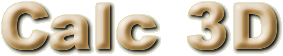## Screenshots

Click the small images to view a full-size screen shot.Redattore With the editor there is not only the standard functionality (saving, printing, printpreview, font, table, paragraph), but also the ability of integation and easy functions.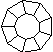Funzione del diagramma Plot functions f(x)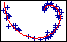Diagramma polare Example for polar diagram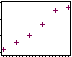Best fit Statistics: best fit, fast fourier transformation, histogram, smooth,....3D-Data Plot a function with 2 variables in a 3D-View and rotate. The rotation can be achieved by mouse interaction. It can be viewed from any direction, can be zoomed and panned.Optionally, axes and/or a bounding box may be displayed.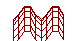Diagramma f(x,y) 3 dimensional Gridnet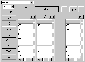Vettori For vectors basic operations (+-*/), crossproduct, spar product, unit vector and angle between two vectors can be evaluated. The professional version has more dimensions and a random generator.Matrici For matrices basic operations (+-*/), inverse, determinante, rotation matrix and multiplication with a vector can be evaluated. The professional version can also split in 2 diagonal matrices, sum the diagonal elements, transpose the matrix, has more dimensions and a random generator.2 Punti For objects like point, line, plane and sphere distances, intersections, volume, area of squeres, area of a triangle can be calculated. In this example the distance of 2 Points is calculated.Linea - cerchio In this example the intersection between a circle and a line is calculated.Piano - linea The combination of a line and a plane implies, that the line is in the plane, or intersects in a point, or they dont intersect. This example shows the intersection of line and a plane.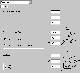Coordinate Cartesian coordinates, spherical coordinates und cylindrical coordinates can be transformed into each other.Numeri complessi Calculating with complex numbers. Elementar and trigonometric functions.Quaternions Calculating with quaternions. Elementar and trigonometric functions.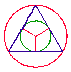Regular Polygon Paint regular polygons with inner circle, outer circle, radius, diagonals. Parameters like area, perimeter and the angles in center and corner are calculated. Because the number of sides is variable, this is good for explaining the area and perimeter of a circle.Circles Circle: Sector, Segment, Intersection of 2 circles.